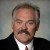# Is it Possible to Determine a Corporate-wide Sigma Level? How Can the Sigma Level be Calculated?

ByDr. Mikel Harry

So as to establish a corporate-level sigma value (per se), it is generally advisable to follow an established analytical model. Of course, any such model must be conducive to the hierarchical aggregation (pooling) of capability information and data. Generally speaking, the more common approaches for realizing this aim are inclusive of, but not limited to: 1) the DPU system, 2) the Z method and 3) the yield procedure, just to mention the most popular.

It is anticipated that the following “cookbooks” will serve to generally illustrate these approaches and analytically demonstrate how they can be pooled to establish an overall sigma value. Clearly recognize that Case A is related to a product that is manifestly different from the product specified in Case B.  As may be apparent, Case C constitutes the integration of cases A and B, respectively.

### Case A: Initiated With Short-term Opportunity-level Quality Information

Step 1: Declare the short-term standard normal deviate
Z.st.d =  3.50  = Input

Step 2: Declare the mean shift
Z.shift.d =  1.50  = Input

Step 3: Approximate the long-term standard normal deviate
Z.lt.a =   2.00  =Z.st.d – Z.shift.d

Step 4: Compute the equivalent long-term throughput-yield
Y.tp.lt.e =  0.977250  =normsdist(Z.lt.a)

Step 5: Declare the unit-level defect opportunity count
O.u.d =  2,400  = Input

Step 6: Compute the equivalent long-term rolled-throughput yield
Y.rt.lt.e =  1.03172E-24  =Y.tp.lt.e^O.u.d

Step 7: Compute the equivalent long-term defects per unit
DPU.lt.e =  55.23  = -ln(Y.rt.lt.e)

### Case B: Initiated With Long-term Unit-level Quality Information

Step 1: Declare the long-term defects per unit
DPU.lt.d =  55.23  = Input

Step 2: Compute the equivalent long-term rolled-throughput yield
Y.rt.lt.e =  1.03172E-24  =exp(-DPU.lt.d)

Step 3: Declare the unit-level defect opportunity count
O.u.d. =  2,400  = Input

Step 4: Compute the equivalent long-term throughput-yield
Y.tp.lt.e =  0.977250  =Y.rt.lt.e^(1 / O.u.d)

Step 5: Compute the equivalent long-term standard normal deviate
Z.lt.e =  2.00  =normsinv(Y.tp.lt.e)

Step 6: Declare the mean shift (per opportunity)
Z.shift.d =  1.50  = Input

Step 7: Approximate the short-term standard normal deviate
Z.st.a =  3.50  =Z.lt.e + Z.shift.d

Handpicked Content:   Core Set of Effectiveness Metrics for Software and IT

### Case C: Pooled Results for Cases A and B

Step 1: Calculate the total long-term defects per unit
TDPU.lt.=  110.462  =DPU.lt.e + DPU.lt.d

Step 2: Calculate the total unit-level opportunity count
TO.u =   4,800  = O.u.d.A + O.u.d.B

Step 3: Normalize the long-term unit-level defects per opportunity
DPO.lt.n =  0.023013  =TDPU.lt / TO.u

Step 4: Normalize the throughput-yield
Y.tp.n =  0.977250  =exp(-DPO.lt.n)

Step 5: Normalize the long-term standard normal deviate
Z.lt.n =  2.00  =normsinv(Y.tp.n)

Step 6: Declare the mean shift
Z.shift.d =  1.50  = Input

Step 7: Normalize the short-term standard normal deviate
Z.st.n =  3.50  =Z.lt.n + Z.shift.d